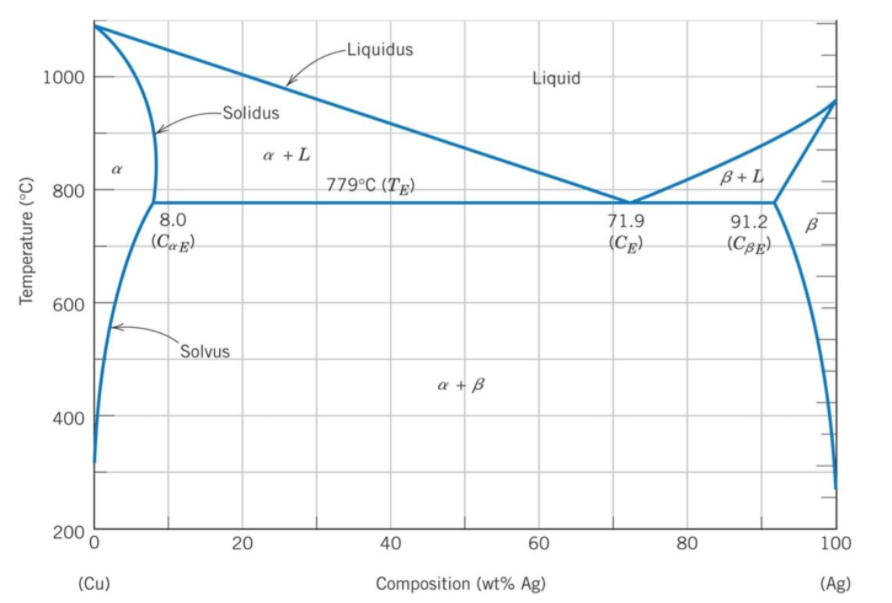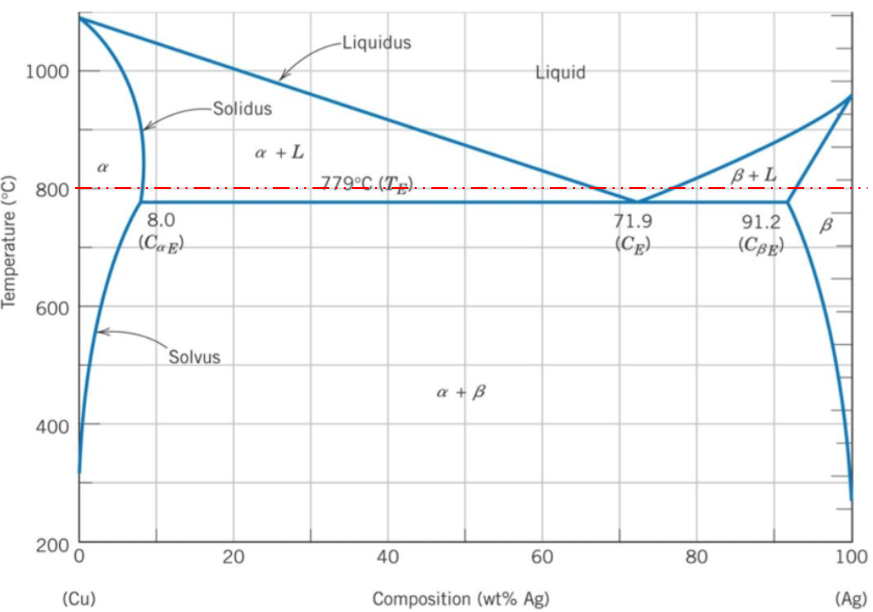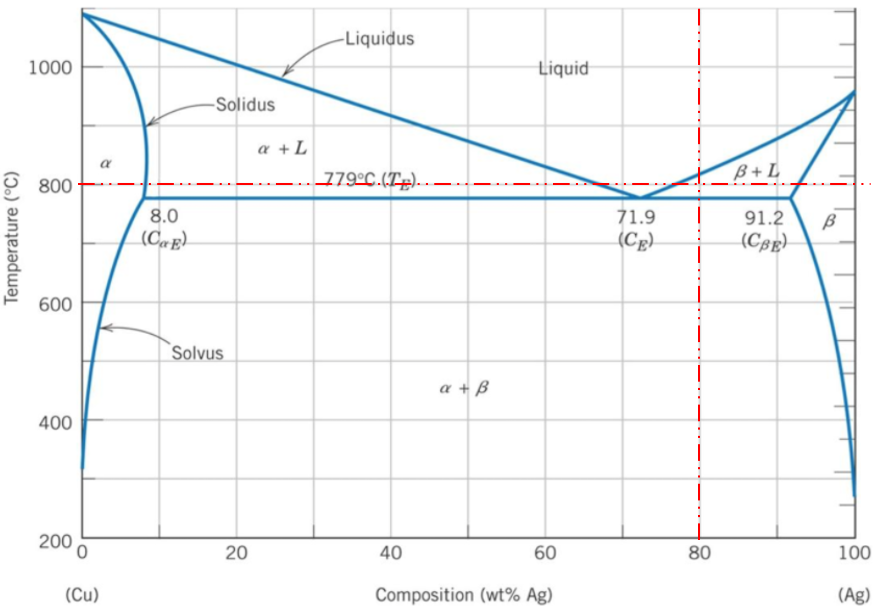## Phase Diagram

An alloy that is 80% silver by weight is fully melted and allowed to slowly cool. Using the binary phase diagram, what phase(s) are present at 800°C?HintHint 2A binary phase diagram shows the phases (solid, liquid etc) formed by mixing two different elements over a range of temperatures. The phase diagram's x-axis represents the alloy's composition weight of silver. The y-axis represents the alloy's temperature. The 80% silver by weight intersects a 800°C temperature in the $$\beta +L$$ zone, meaning $$\beta +L$$ phases are present.$$\beta +L$$\$